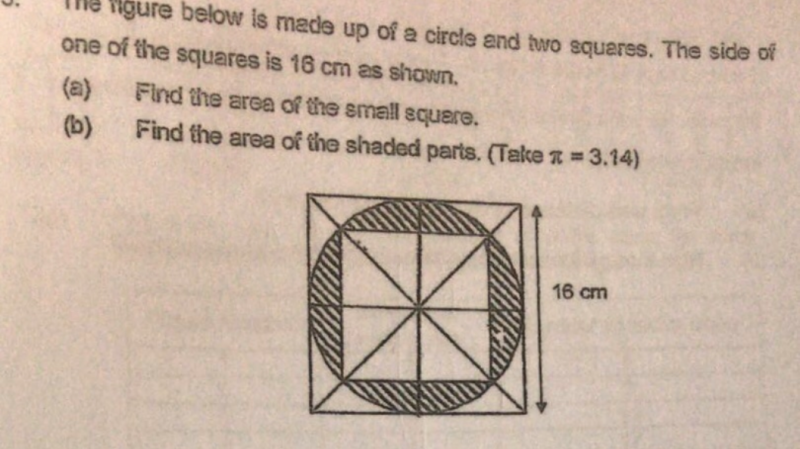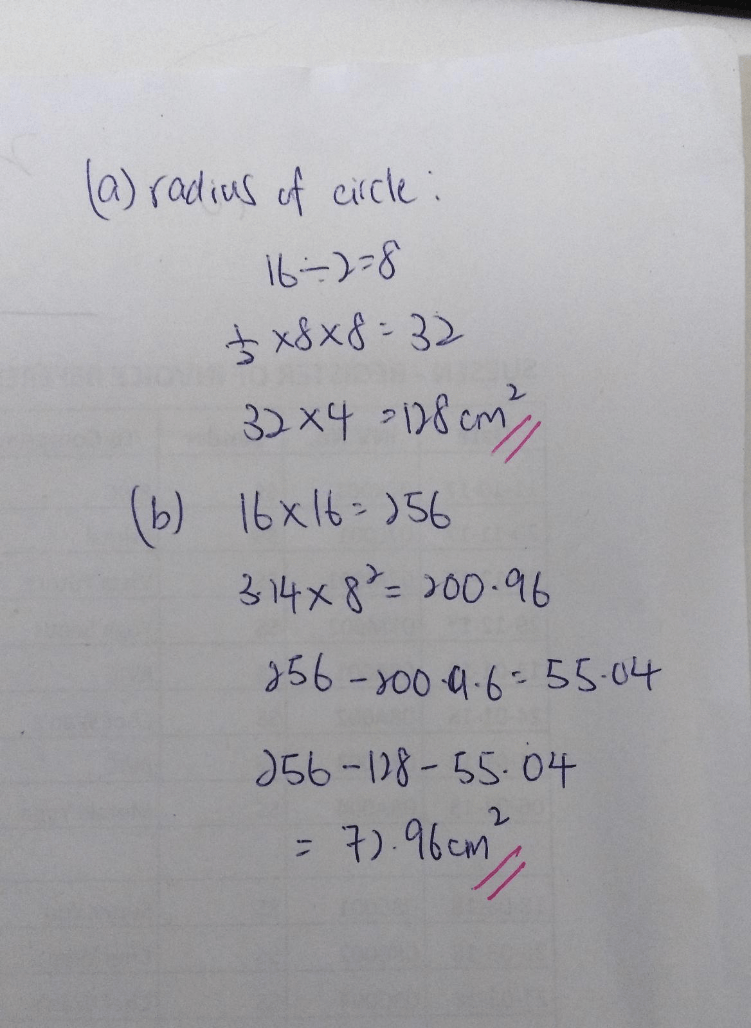# Question(a)
radius of circle = 16/2 = 8
Rotate the inner square 45 degrees,
area of the small squares = 4 x (1/2) x 8 x 8 = 128

(b)
area of shaded parts = 3.14 x 8 x 8 – 128 = 72.96

Ans : (a) 128 sq cm; (b) 72.96 sq cm.

0 Replies 1 Like